Saurabh.jaju2 — Published On April 10, 2017 and Last Modified On February 7th, 2023

## Introduction

Time Series forecasting & modeling plays an important role in data analysis. Time series analysis is a specialized branch of statistics used extensively in fields such as Econometrics & Operation Research. This skilltest was conducted to test your knowledge of time series concepts.

A total of 1094 people registered for this skill test. The test was designed to test you on the basic & advanced level of time series. If you are one of those who missed out on this skill test, here are the questions and solutions. You missed on the real time test, but can read this article to find out how many could have answered correctly.

Here are the leaderboard ranking for all the participants.

## Overall Scores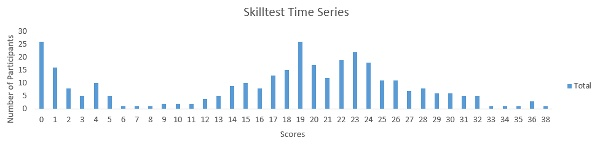You can access the scores here. More than 300 people participated in the skill test and the highest score obtained was 38. Here are a few statistics about the distribution.

Mean Score: 17.13

Median Score: 19

Mode Score: 19

## Useful Resources

A Complete Tutorial on Time Series Modeling in R

A comprehensive beginner’s guide to create a Time Series Forecast (with Codes in Python)

1) Which of the following is an example of time series problem?
1. Estimating number of hotel rooms booking in next 6 months.
2. Estimating the total sales in next 3 years of an insurance company.
3. Estimating the number of calls for the next one week.

A) Only 3
B) 1 and 2
C) 2 and 3
D) 1 and 3
E) 1,2 and 3

Solution: (E)

All the above options have a time component associated.

2) Which of the following is not an example of a time series model?

A) Naive approach
B) Exponential smoothing
C) Moving Average
D)None of the above

Solution: (D)

Naïve approach: Estimating technique in which the last period’s actuals are used as this period’s forecast, without adjusting them or attempting to establish causal factors. It is used only for comparison with the forecasts generated by the better (sophisticated) techniques.

In exponential smoothing, older data is given progressively-less relative importance whereas newer data is given progressively-greater importance.

In time series analysis, the moving-average (MA) model is a common approach for modeling univariate time series. The moving-average model specifies that the output variable depends linearly on the current and various past values of a stochastic (imperfectly predictable) term.

3) Which of the following can’t be a component for a time series plot?

A) Seasonality
B) Trend
C) Cyclical
D) Noise
E)  None of the above

Solution: (E)

A seasonal pattern exists when a series is influenced byseasonal factors (e.g., the quarter of the year, the month, or day of the week). Seasonality is always of a fixed and known period. Hence, seasonal time series are sometimes called periodic time series

Seasonality is always of a fixed and known period. A cyclic pattern exists when data exhibit rises and falls that are not of fixed period.

Trend is defined as the ‘long term’ movement in a time series without calendar related and irregular effects, and is a reflection of the underlying level. It is the result of influences such as population growth, price inflation and general economic changes. The following graph depicts a series in which there is an obvious upward trend over time.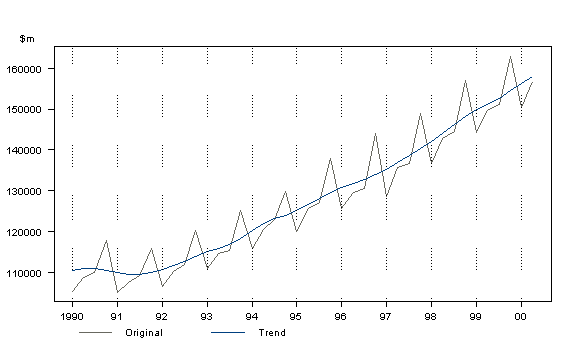Quarterly Gross Domestic Product

Noise: In discrete time, white noise is a discrete signal whose samples are regarded as a sequence of serially uncorrelated random variables with zero mean and finite variance.

Thus all of the above mentioned are components of a time series.

4) Which of the following is relatively easier to estimate in time series modeling?

A) Seasonality
B) Cyclical
C) No difference between Seasonality and CyclicalSolution: (A)As we seen in previous solution, as seasonality exhibits fixed structure; it is easier to estimate.

5) The below time series plot contains both Cyclical and Seasonality component.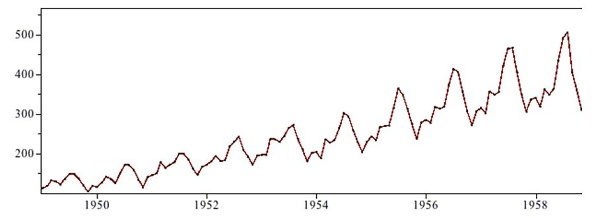A) TRUE
B) FALSESolution: (B)There is a repeated trend in the plot above at regular intervals of time and is thus only seasonal in nature.6) Adjacent observations in time series data (excluding white noise) are independent and identically distributed (IID).

A) TRUE

B) FALSE

Solution: (B)

Clusters of observations are frequently correlated with increasing strength as the time intervals between them become shorter. This needs to be true because in time series forecasting is done based on previous observations and not the currently observed data unlike classification or regression.

7) Smoothing parameter close to one gives more weight or influence to recent observations over the forecast.

A) TRUE
B) FALSE

Solution: (A)

It may be sensible to attach larger weights to more recent observations than to observations from the distant past. This is exactly the concept behind simple exponential smoothing. Forecasts are calculated using weighted averages where the weights decrease exponentially as observations come from further in the past — the smallest weights are associated with the oldest observations:

y^T+1|T=αyT+α(1−α)yT−1+α(1−α)2yT−2+⋯,(7.1)

where 0≤α≤10≤α≤1 is the smoothing parameter. The one-step-ahead forecast for time T+1T+1 is a weighted average of all the observations in the series y1,…,yT. The rate at which the weights decrease is controlled by the parameter αα.

8) Sum of weights in exponential smoothing is _____.

A) <1
B)  1
C)  >1
D) None of the above

Solution: (B)

Table 7.1 shows the weights attached to observations for four different values of αα when forecasting using simple exponential smoothing. Note that the sum of the weights even for a small αα will be approximately one for any reasonable sample size.

 Observation α=0.2 α=0.4 α=0.6 α=0.8 yT 0.2 0.4 0.6 0.8 yT−1 0.16 0.24 0.24 0.16 yT−2 0.128 0.144 0.096 0.032 yT−3 0.102 0.0864 0.0384 0.0064 yT−4 (0.2)(0.8) (0.4)(0.6) (0.6)(0.4) (0.8)(0.2) yT−5 (0.2)(0.8) (0.4)(0.6) (0.6)(0.4) (0.8)(0.2)

9) The last period’s forecast was 70 and demand was 60. What is the simple exponential smoothing forecast with alpha of 0.4 for the next period.

A) 63.8
B) 65
C) 62
D) 66

Solution: (D)

Yt-1= 70

St-1= 60

Alpha = 0.4

Substituting the values we get

0.4*60 + 0.6*70= 24 + 42= 66

10) What does autocovariance measure?

A) Linear dependence between multiple points on the different series observed at different times
B)Quadratic dependence between two points on the same series observed at different times
C) Linear dependence between two points on different series observed at same time
D) Linear dependence between two points on the same series observed at different times

Solution: (D)

Option D is the definition of autocovariance.

11) Which of the following is not a necessary condition for weakly stationary time series?

A) Mean is constant and does not depend on time
B) Autocovariance function depends on s and t only through their difference |s-t| (where t and s are moments in time)
C) The time series under considerations is a finite variance process
D) Time series is Gaussian

Solution: (D)

A Gaussian time series implies stationarity is strict stationarity.

12) Which of the following is not a technique used in smoothing time series?

A) Nearest Neighbour Regression
B)  Locally weighted scatter plot smoothing
C) Tree based models like (CART)
D) Smoothing Splines

Solution: (C)

Time series smoothing and ﬁltering can be expressed in terms of local regression models. Polynomials and regression splines also provide important techniques for smoothing. CART based models do not provide an equation to superimpose on time series and thus cannot be used for smoothing. All the other techniques are well documented smoothing techniques.

13) If the demand is 100 during October 2016, 200 in November 2016, 300 in December 2016, 400 in January 2017. What is the 3-month simple moving average for February 2017?

A) 300
B) 350
C) 400

Solution: (A)

X`= (xt-3 + xt-2 + xt-1 ) /3

(200+300+400)/ 3 = 900/3 =300

14) Looking at the below ACF plot, would you suggest to apply AR or MA in ARIMA modeling technique?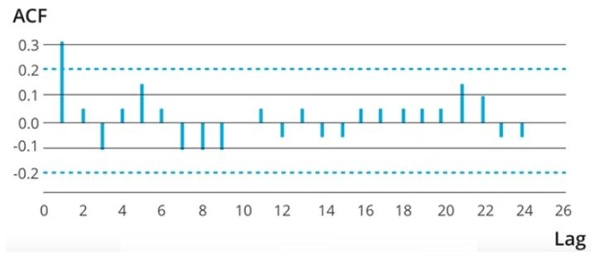A) AR
B) MA
C) Can’t SaySolution: (A)MA model is considered in the following situation, If the autocorrelation function (ACF) of the differenced series displays a sharp cutoff and/or the lag-1 autocorrelation is negative–i.e., if the series appears slightly “overdifferenced”–then consider adding an MA term to the model. The lag beyond which the ACF cuts off is the indicated number of MA terms.But as there are no observable sharp cutoffs the AR model must be preffered.

15) Suppose, you are a data scientist at Analytics Vidhya. And you observed the views on the articles increases during the month of Jan-Mar. Whereas the views during Nov-Dec decreases.

Does the above statement represent seasonality?A) TRUE
B) FALSE
C) Can’t SaySolution: (A)Yes this is a definite seasonal trend as there is a change in the views at particular times.Remember, Seasonality is a presence of variations at specific periodic intervals.

16) Which of the following graph can be used to detect seasonality in time series data?

1. Multiple box
2. AutocorrelationA) Only 1
B) Only 2
C) 1 and 2
D)  None of theseSolution: (C)Seasonality is a presence of variations at specific periodic intervals.The variation of distribution can be observed in multiple box plots. And thus seasonality can be easily spotted. Autocorrelation plot should show spikes at lags equal to the period.

17) Stationarity is a desirable property for a time series process.

A) TRUE
B) FALSESolution: (A)When the following conditions are satisfied then a time series is stationary.

1. Mean is constant and does not depend on time
2. Autocovariance function depends on s and t only through their difference |s-t| (where t and s are moments in time)
3. The time series under considerations is a finite variance process

These conditions are essential prerequisites for mathematically representing a time series to be used for analysis and forecasting. Thus stationarity is a desirable property.

18) Suppose you are given a time series dataset which has only 4 columns (id, Time, X, Target).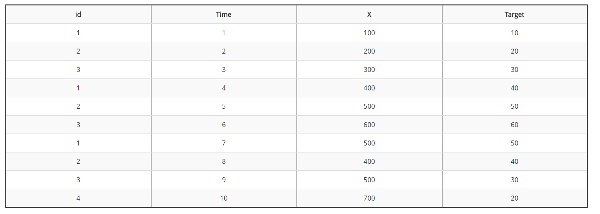What would be the rolling mean of feature X if you are given the window size 2?Note: X column represents rolling mean.A)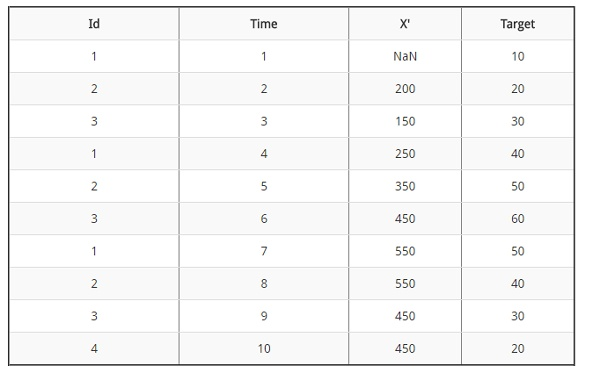B)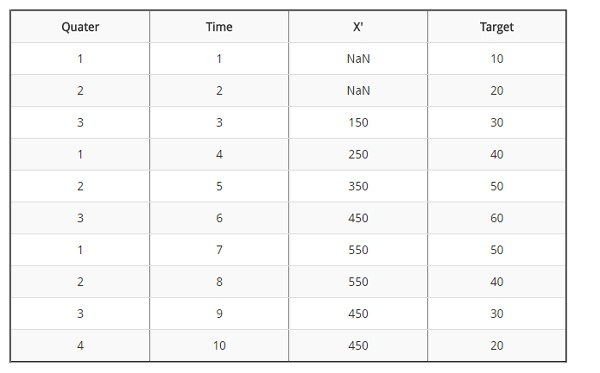C)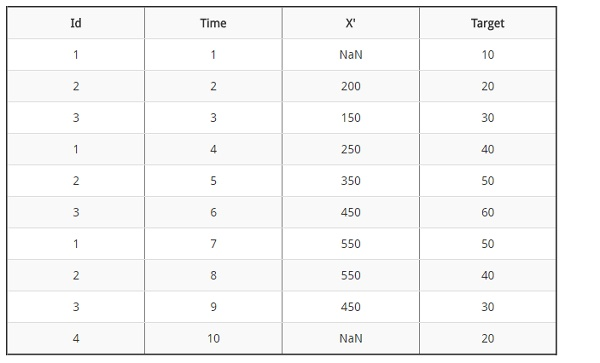D) None of the above

Solution: (B)

X`= xt-2 + xt-1 /2

Based on the above formula: (100 +200) /2 =150; (200+300)/2 = 250 and so on.

19) Imagine, you are working on a time series dataset. Your manager has asked you to build a highly accurate model. You started to build two types of models which are given below.

Model 1: Decision Tree modelModel 2: Time series regression model

At the end of evaluation of these two models, you found that model 2 is better than model 1. What could be the possible reason for your inference?

A) Model 1 couldn’t map the linear relationship as good as Model 2
B) Model 1 will always be better than Model 2
C) You can’t compare decision tree with time series regression
D) None of these

Solution: (A)

A time series model is similar to a regression model. So it is good at finding simple linear relationships. While a tree based model though efficient will not be as good at finding and exploiting linear relationships.

20) What type of analysis could be most effective for predicting temperature on the following type of data.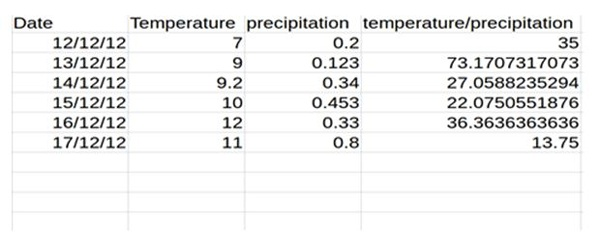A) Time Series Analysis
B) Classification
C)  Clustering
D) None of the above

Solution: (A)

The data is obtained on consecutive days and thus the most effective type of analysis will be time series analysis.

21) What is the first difference of temperature / precipitation variable?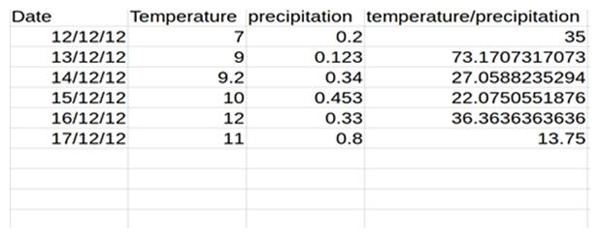A) 15,12.2,-43.2,-23.2,14.3,-7
B) 38.17,-46.11,-4.98,14.29,-22.61
C) 35,38.17,-46.11,-4.98,14.29,-22.61
D) 36.21,-43.23,-5.43,17.44,-22.61

Solution: (B)

73.17-35 = 38.17

27.05-73.17 = – 46.11 and so on..

13.75 – 36.36 = -22.61

22) Consider the following set of data:

{23.32 32.33 32.88 28.98 33.16 26.33 29.88 32.69 18.98 21.23 26.66 29.89}

What is the lag-one sample autocorrelation of the time series?

A) 0.26
B) 0.52
C) 0.13
D) 0.07

Solution: (C)

ρˆ1 = PT t=2(xt−1−x¯)(xt−x¯) PT t=1(xt−x¯) 2

= (23.32−x¯)(32.33−x¯)+(32.33−x¯)(32.88−x¯)+··· PT t=1(xt−x¯) 2

= 0.130394786

Where x¯ is the mean of the series which is 28.0275

23) Any stationary time series can be approximately the random superposition of sines and cosines oscillating at various frequencies.

A) TRUE
B) FALSESolution: (A)A weakly stationary time series, xt, is a ﬁnite variance process such that

• The mean value function, µt, is constant and does not depend on time t, and (ii) the autocovariance function, γ(s,t), deﬁned in depends on s and t only through their diﬀerence |s−t|.

random superposition of sines and cosines oscillating at various frequencies is white noise. white noise is weakly stationary or stationary. If the white noise variates are also normally distributed or Gaussian, the series is also strictly stationary.

24) Autocovariance function for weakly stationary time series does not depend on _______ ?

A)  Separation of xs and xt
B) h = | s – t |
C) Location of point at a particular timeSolution: (C)By definition of weak stationary time series described in previous question.

25) Two time series are jointly stationary if _____ ?

A) They are each stationary
B) Cross variance function is a function only of lag h

A) Only A
B) Both A and B

Solution: (D)

Joint stationarity is defined based on the above two mentioned conditions.

26) In autoregressive models _______ ?

A) Current value of dependent variable is influenced by current values of independent variables
B) Current value of dependent variable is influenced by current and past values of independent variables
C) Current value of dependent variable is influenced by past values of both dependent and independent variables
D) None of the aboveSolution: (C)Autoregressive models are based on the idea that the current value of the series, xt, can be explained as a function of p past values, xt−1,xt−2,…,xt−p, where p determines the number of steps into the past needed to forecast the current value. Ex. xt = xt−1 −.90xt−2 + wt,Where xt-1  and xt-2 are past values of dependent variable and wt the white noise can represent values of independent values.

The example can be extended to include multiple series analogous to multivariate linear regression.

27) For MA (Moving Average) models the pair σ = 1 and θ = 5 yields the same autocovariance function as the pair σ = 25 and θ = 1/5.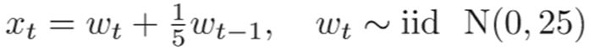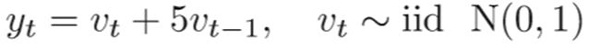A) TRUE
B) FALSESolution: (A)True, because autocovariance is invertible for MA modelsnote that for an MA(1) model, ρ(h) is the same for θ and 1 /θ

try 5 and 1 5, for example. In addition, the pair σ2 w = 1 and θ = 5 yield the same autocovariance function as the pair σ2 w = 25 and θ = 1/5.

28) How many AR and MA terms should be included for the time series by looking at the above ACF and PACF plots?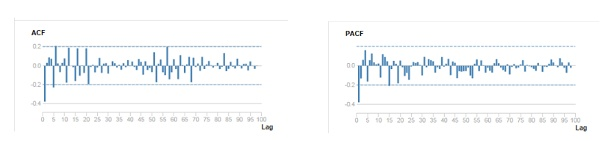A) AR (1) MA(0)
B) AR(0)MA(1)
C) AR(2)MA(1)
D) AR(1)MA(2)
E) Can’t Say

Solution: (B)

Strong negative correlation at lag 1 suggest MA and there is only 1 significant lag. Read this article for a better understanding.

29) Which of the following is true for white noise?

A) Mean =0
B) Zero autocovariances
C) Zero autocovariances except at lag zero
D) Quadratic VarianceSolution: (C)A white noise process must have a constant mean, a constant variance and no autocovariance structure (except at lag zero, which is the variance).

30) For the following MA (3) process yt = μ + Εt + θ1Εt-1 + θ2Εt-2 + θ3Εt-3 , where σt is a zero mean white noise process with variance σ2

A) ACF = 0 at lag 3
B) ACF =0 at lag 5
C) ACF =1 at lag 1
D) ACF =0 at lag 2
E) ACF = 0 at lag 3 and at lag 5Solution: (B)Recall that an MA(q) process only has memory of length q. This means that all of the autocorrelation coefficients will have a value of zero beyond lag q. This can be seen by examining the MA equation, and seeing that only the past q disturbance terms enter into the equation, so that if we iterate this equation forward through time by more than q periods, the current value of the disturbance term will no longer affect y. Finally, since the autocorrelation function at lag zero is the correlation of y at time t with y at time t (i.e. the correlation of y_t with itself), it must be one by definition.

31) Consider the following AR(1) model with the disturbances having zero mean and unit variance.
yt= 0.4 + 0.2yt-1+ut
The (unconditional) variance of y will be given by ?

A)  1.5
B) 1.04
C) 0.5
D)  2

Solution: (B)

Variance of the disturbances divided by (1 minus the square of the autoregressive coefficient

Which in this case is : 1/(1-(0.2^2))= 1/0.96= 1.041

32) The pacf (partial autocorrelation function) is necessary for distinguishing between ______ ?

A) An AR and MA model is_solution: False
B)  An AR and an ARMA is_solution: True
C) An MA and an ARMA is_solution: False
D) Different models from within the ARMA family

Solution: (B)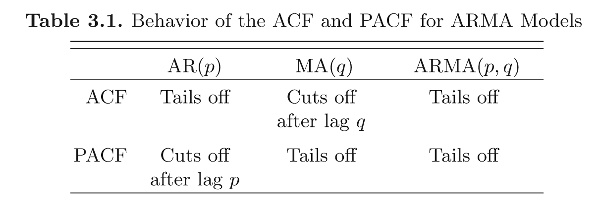33) Second differencing in time series can help to eliminate which trend?

B) Linear Trend
C) Both A & B
D) None of the aboveSolution:  (A)The ﬁrst diﬀerence is denoted as ∇xt = xt −xt−1. (1)As we have seen, the ﬁrst diﬀerence eliminates a linear trend. A second diﬀerence, that is, the diﬀerence of (1), can eliminate a quadratic trend, and so on.

34) Which of the following cross validation techniques is better suited for time series data?

A)  k-Fold Cross Validation
B) Leave-one-out Cross Validation
C) Stratified Shuffle Split Cross Validation
D) Forward Chaining Cross Validation

Solution: (D)

Time series is ordered data. So the validation data must be ordered to. Forward chaining ensures this. It works as follows:

• fold 1 : training , test 
• fold 2 : training [1 2], test 
• fold 3 : training [1 2 3], test 
• fold 4 : training [1 2 3 4], test 
• fold 5 : training [1 2 3 4 5], test 

35) BIC penalizes complex models more strongly than the AIC.

A) TRUE
B) FALSESolution: (A)AIC = -2*ln(likelihood) + 2*k,BIC = -2*ln(likelihood) + ln(N)*k,where:

k = model degrees of freedom

N = number of observations

At relatively low N (7 and less) BIC is more tolerant of free parameters than AIC, but less tolerant at higher N (as the natural log of N overcomes 2).

36) The figure below shows the estimated autocorrelation and partial autocorrelations of a time series of n = 60 observations. Based on these plots, we should.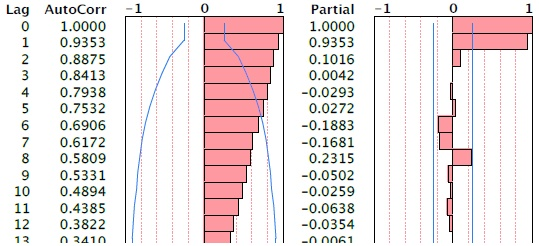A) Transform the data by taking logs
B) Difference the series to obtain stationary data
C) Fit an MA(1) model to the time series

Solution: (B)

The autocorr shows a definite trend and partial autocorrelation shows a choppy trend, in such a scenario taking a log would be of no use. Differencing the series to obtain a stationary series is the only option.

Question Context (37-38)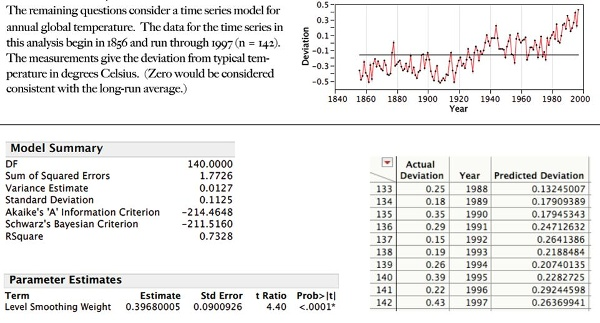37) Use the estimated exponential smoothening given above and predict temperature for the next 3 years (1998-2000)

These results summarize the fit of a simple exponential smooth to the time series.

A) 0.2,0.32,0.6
B) 0.33, 0.33,0.33
C) 0.27,0.27,0.27
D) 0.4,0.3,0.37

Solution: (B)

The predicted value from the exponential smooth is the same for all 3 years, so all we need is the value for next year. The expression for the smooth is

smootht = α yt + (1 – α) smooth t-1

Hence, for the next point, the next value of the smooth (the prediction for the next observation) is

smoothn = α yn + (1 – α) smooth n-1

= 0.3968*0.43 + (1 – 0.3968)* 0.3968

= 0.3297

38) Find 95% prediction intervals for the predictions of temperature in 1999.

These results summarize the fit of a simple exponential smooth to the time series.A) 0.3297 2 * 0.1125
B) 0.3297 2 * 0.121
C) 0.3297 2 * 0.129
D) 0.3297 2 * 0.22Solution: (B)The sd of the prediction errors is1 period out 0.1125

2 periods out 0.1125 sqrt(1+α2) = 0.1125 * sqrt(1+ 0.39682) ≈ 0.121

39) Which of the following statement is correct?

1. If autoregressive parameter (p) in an ARIMA model is 1, it means that there is no auto-correlation in the series.
2. If moving average component (q) in an ARIMA model is 1, it means that there is auto-correlation in the series with lag 1.
3. If integrated component (d) in an ARIMA model is 0, it means that the series is not stationary.

A) Only 1
B) Both 1 and 2
C) Only 2
D)  All of the statements

Solution: (C)

Autoregressive component: AR stands for autoregressive.  Autoregressive parameter is denoted by p.  When p =0, it means that there is no auto-correlation in the series.  When p=1, it means that the series auto-correlation is till one lag.

Integrated: In ARIMA time series analysis, integrated is denoted by d.  Integration is the inverse of differencing.  When d=0, it means the series is stationary and we do not need to take the difference of it.  When d=1, it means that the series is not stationary and to make it stationary, we need to take the first difference.  When d=2, it means that the series has been differenced twice.  Usually, more than two time difference is not reliable.

Moving average component: MA stands for moving the average, which is denoted by q.  In ARIMA, moving average q=1 means that it is an error term and there is auto-correlation with one lag.

40) In a time-series forecasting problem, if the seasonal indices for quarters 1, 2, and 3 are 0.80, 0.90, and 0.95 respectively. What can you say about the seasonal index of quarter 4?

A) It will be less than 1
B)  It will be greater than 1
C) It will be equal to 1
D) Seasonality does not exist
E) Data is insufficient

Solution: (B)

The seasonal indices must sum to 4, since there are 4 quarters. .80 + .90 + .95 = 2.65, so the seasonal index for the 4th quarter must be 1.35 so B is the correct answer.

## End Notes

If you missed out on this competition, make sure you complete in the ones coming up shortly. We are giving cash prizes worth \$10,000+ during the month of April 2017.

If you have any questions or doubts feel free to post them below.

#### Check out all the upcoming skilltests here.### Our Top Authors

•